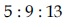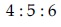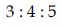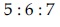#### If the lengths of the sides of a triangle are in A.P. and the greatest angle is double the smallest, then a ratio of lengths of the sides of this triangle is :  Option 1)Option 2)Option 3)Option 4)Letbe side ofis smallest angle

Three angles are,Given,Use sine rule.Option 1)Option 2)Option 3)Option 4)